Tamilnadu State Board New Syllabus Samacheer Kalvi 12th Business Maths Guide Pdf Chapter 1 Applications of Matrices and Determinants Miscellaneous Problems Text Book Back Questions and Answers, Notes.

## Tamilnadu Samacheer Kalvi 12th Business Maths Solutions Chapter 1 Applications of Matrices and Determinants Miscellaneous Problems

Question 1.
Find the rank of the matrix
A = $$\left(\begin{array}{cccc} 1 & -3 & 4 & 7 \\ 9 & 1 & 2 & 0 \end{array}\right)$$
Solution:
$$\left(\begin{array}{cccc} 1 & -3 & 4 & 7 \\ 9 & 1 & 2 & 0 \end{array}\right)$$
The order of A is 2 × 4
∴ P(A) ≤ 2
Let us transform the matrix A to an echelon formThe number of non-zero rows is 2
∴ P(A) = 2Question 2.
Find the rank of the matrix
A = $$\left[\begin{array}{cccc} -2 & 1 & 3 & 4 \\ 0 & 1 & 1 & 2 \\ 1 & 3 & 4 & 7 \end{array}\right]$$
Solution:
A = $$\left[\begin{array}{cccc} -2 & 1 & 3 & 4 \\ 0 & 1 & 1 & 2 \\ 1 & 3 & 4 & 7 \end{array}\right]$$
The order of A is 3 × 4
∴ P(A) < 3
Let us transform the matrix A to an echelon formThe number of non-zero rows = 3
∴ P(A) = 3Question 3.
Find the rank of the matrix
A = $$\left[\begin{array}{llll} 4 & 5 & 2 & 2 \\ 3 & 2 & 1 & 6 \\ 4 & 4 & 8 & 0 \end{array}\right]$$
Solution:
A = $$\left[\begin{array}{llll} 4 & 5 & 2 & 2 \\ 3 & 2 & 1 & 6 \\ 4 & 4 & 8 & 0 \end{array}\right]$$
The order of A is 3 × 4
∴ p(A) < 3
Let us transform the matrix A to an echelon form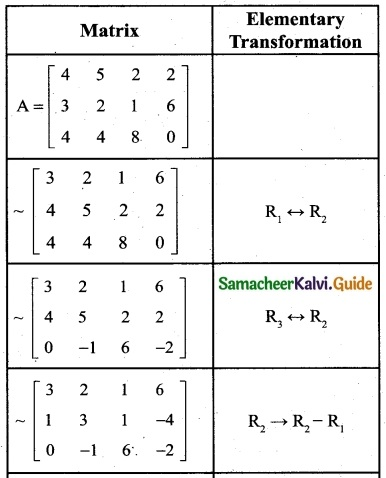The last equivalent matrix is in the echelon form.
Number of non-zero rows = 3
∴ P(A) = 3Question 4.
Examine the consistency of the system of equations;
x + y + z = 7, x + 2y + 3z = 18, y + 2z = 6
Solution:
x + y + z = 7
x + 2y + 3z = 18
y + 2z = 6
The matrix form of the equationsHere p(A) ≠ p(A, B)
∴ The given system is inconsistent and has no solution.

Question 5.
Find k if the equations 2x + 3y – z = 5, 3x – y + 4z = 2, x + 7y – 6z = k are consistent.
Solution:
2x + 3y – z = 5
3x – y + 4z = 2
x + 7y – 6z = k
The matrix form of these equations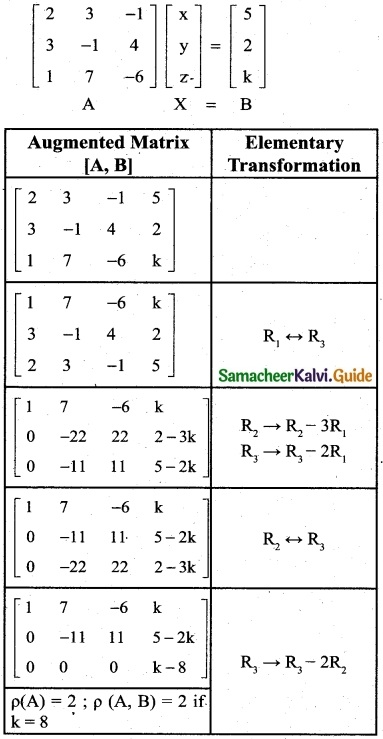Obviously, the last equivalent matrix is in the ech-elon form.
Since the equations are consistent
P(-A) = p(A, B)
p(A) = 2 and p (A, B) = 2 then
k = 8Question 6.
Find k if the equations x + y + z = 1, 3x – y – z = 4, x + 5y + 5z = k are inconsistent.
Solution:
x + y + z = 1
3x – y – z = 4
x + 5y + 5z = k
The matrix equation corresponding to the given system isObviously, the last equivalent matrix is in the ech-elon form.
Since the equations are inconsistent
p(A) ≠ p (A, B)
Here p(A) = 2 but p(A, B) should not equal to 2
∴ k ≠ 0
The equations are inconsistent when k assume any real value other than 0.Question 7.
Solve the equations x + 2y + z = 7, 2x – y + 2z = 4, x + y – 2z = -1 by using Cramer’s rule.
Solution:
x + 2y + z = 7
2x – y + 2z = 4
x + y – 2z = -1
Here Δ = $$\left|\begin{array}{ccc} 1 & 2 & 1 \\ 2 & -1 & 2 \\ 1 & 1 & -2 \end{array}\right|$$
= 1(2 – 2)-2 (-4 – 2) + 1(2 + 1)
= 1(0) -2 (-6) + 1(3)
= 12 + 3 = 15 ≠ 0
∴ We can apply Cramer’s Rule and the system is consistent and it has unique solution.
Δx = $$\left|\begin{array}{ccc} 7 & 2 & 1 \\ 4 & -1 & 2 \\ -1 & 1 & -2 \end{array}\right|$$
= 7 (2 – 2) -2 (-8 + 2) + 1(4 – 1)
= 7(0)-2 (-6) + 1(3)
= 12 + 3
= 15
Δy = $$\left|\begin{array}{ccc} 1 & 7 & 1 \\ 2 & 4 & 2 \\ 1 & -1 & -2 \end{array}\right|$$
= 1(-8 + 2) -7 (-4 – 2) + 1(-2 – 4)
= 1 (-6) – 7 (-6) + 1 (-6)
= -6 + 42 – 6 = 30
Δz = $$\left|\begin{array}{ccc} 1 & 2 & 7 \\ 2 & -1 & 4 \\ 1 & 1 & -1 \end{array}\right|$$
= 1(1 – 4) -2 (-2 – 4) + 7 (2 + 1)
= 1 (-3) -2 (-6) + 7 (3)
= -3 + 12 + 21 = 30
∴ By cramer’s rule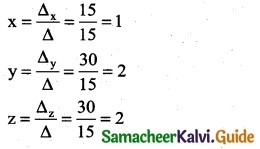∴ The Solution is (x, y, z) = (1, 2, 2)Question 8.
The cost of 2kg. of wheat and 1kg. of sugar is Rs 100. The cost of 3kg. of wheat, 2kg. of sugar and 1kg of rice is Rs 220. Find the cost of each per kg. using Cramer’s rule.
Solution:
Let cost of 1 kg of wheat be x; cost of 1kg of sugar be y and cost of 1 kg of rice be z.
2x + y = 100
x + z = 80
3x + 2y + z = 220
Here Δ = $$\left|\begin{array}{ccc} 2 & 1 & 0 \\ 1 & 0 & 1 \\ 3 & 2 & 1 \end{array}\right|$$
= 2 (0 – 2) -1 (1 – 3)
= – 4 + 2 = -2
≠ 0
∴ We can apply Cramer’s Rule and the system is consistent and it has unique solution.
Δx = $$\left|\begin{array}{ccc} 100 & 1 & 0 \\ 80 & 0 & 1 \\ 220 & 2 & 1 \end{array}\right|$$
= 100 (0 – 2) -1 (80 – 220)
= – 200 -1 (-140)
= – 200 +140
= -60
Δy = $$\left|\begin{array}{ccc} 2 & 100 & 0 \\ 1 & 80 & 0 \\ 3 & 220 & 1 \end{array}\right|$$
= 2 (80 – 220) -100 (1 – 3)
= 2 (-140) – 100 (-2)
= – 280 + 200
= -80
Δx = $$\left|\begin{array}{ccc} 2 & 1 & 100 \\ 1 & 0 & 80 \\ 3 & 2 & 220 \end{array}\right|$$
= 2 (0 -160) -1 (220 – 240) + 100 (2 – 0)
= 2 (-160) -1 (-20) + 100 (2)
= -320 + 20 + 200
= -100
∴ By cramer’s rule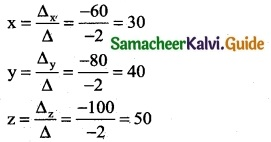The solution is (x, y, z) = (30, 40, 50)
∴ The cost of 1 kg of wheat is Rs 30; the cost of 1 kg of sugar is Rs 40 and the cost of 1 kg of rice is Rs 50.Question 9.
A salesman has the following record of sales during three items A, B, and C, which has different rates of commission.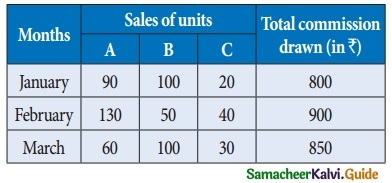Find out the rate of commission on items A, B, and C by using Cramer’s rule.
Solution:
Let x, y, and z be the commission on items A, B, and C respectively.
90x + 100y + 20z = 800 ⇒ 9x + 10y + 2z = 80 ……… (1)
130x + 50y + 40z = 900 ⇒ 13x + 5y + 4z = 90 ………. (2)
60x + 100y + 30z = 850 ⇒ 6x + 10y + 3z = 85 ………. (3)
Here Δ = $$\left|\begin{array}{ccc} 9 & 10 & 2 \\ 13 & 5 & 4 \\ 6 & 10 & 3 \end{array}\right|$$
= 9 (15 – 40) -10 (39 – 24) + 2 (130 – 30)
= 9 (-25) – 10 (15) + 2 (100)
= -225 – 150 + 200
= -175
≠ 0
∴ We can apply Cramer’s Rule and the system is consistent and it has unique solution.
Δx = $$\left|\begin{array}{ccc} 80 & 10 & 2 \\ 90 & 5 & 4 \\ 85 & 10 & 3 \end{array}\right|$$
= 80 (15 – 40) -10 (270 – 340) + 2 (900 – 425)
= 80 (-25) -10 (-70) + 2 (475)
= -2000 + 700 + 950
= -350
Δy = $$\left|\begin{array}{ccc} 9 & 80 & 2 \\ 13 & 90 & 4 \\ 6 & 85 & 3 \end{array}\right|$$
= 9 (270 – 340) – 80 (39 – 24) + 2 (1105 – 540)
= 9 (-70)-80 (15) + 2 (565)
= -630 – 1200 + 1130
= -700
Δz = $$\left|\begin{array}{ccc} 9 & 10 & 80 \\ 13 & 5 & 90 \\ 6 & 10 & 85 \end{array}\right|$$
= 9 (425 – 900) -10 (1105 – 540) + 80 (130 – 30)
= 9 (-475) -10 (565) + 80 (100)
= – 4275 – 5650 + 8000
= -1925
∴ By cramer’s ruleThe solution is (x, y, z) = (2, 4, 11)
∴ The rates of commission for A, B and C are Rs 2, Rs 4 and Rs 11 respectively.Question 10.
The subscription department of a magazine sends out a letter to a large mailing list inviting subscriptions for the magazine. Some of the people receiving this letter already subscribe to the magazine while others do not. From this mailing list, 60% of those who already subscribe will subscribe again while 25% of those who do not now subscribe will subscribe. In the last letter, it was found that 40% of those receiving it ordered a subscription. What percent of those receiving the current letter can be expected to order a subscription?
Solution:
The transition probability matrix is S∴ 39% of those receiving the current letter can be expected to order a subscription.